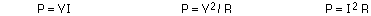## Power and Alternating Current (AC)

7-10-00

Sections 18.5 - 18.8

### Electric power

Power is the rate at which work is done. It has units of Watts. 1 W = 1 J/s

Electric power is given by the equations:The power supplied to a circuit by a battery is calculated using P = VI.

Batteries and power supplies supply power to a circuit, and this power is used up by motors as well as by anything that has resistance. The power dissipated in a resistor goes into heating the resistor; this is know as Joule heating. In many cases, Joule heating is wasted energy. In some cases, however, Joule heating is exploited as a source of heat, such as in a toaster or an electric heater.

The electric company bills not for power but for energy, using units of kilowatt-hours.

1 kW-h = 3.6 x 106 J

One kW-h typically costs about 10 cents, which is really quite cheap. It does add up, though. The following equation gives the total cost of operating something electrical:

Cost = (Power rating in kW) x (number of hours it's running) x (cost per kW-h)

An example...if a 100 W light bulb is on for two hours each day, and energy costs \$0.10 per kW-h, how much does it cost to run the bulb for a month?

Cost = 0.1 kW x 60 hours x \$0.1/kW-h = \$0.6, or 60 cents.

Try this at home - figure out the monthly cost of using a particular appliance you use every day. Possibilities include hair dryers, microwaves, TV's, etc. The power rating of an appliance like a TV is usually written on the back, and if it doesn't give the power it should give the current. Anything you plug into a wall socket runs at 120 V, so if you know that and the current you can figure out how much power it uses.

The cost for power that comes from a wall socket is relatively cheap. On the other hand, the cost of battery power is much higher. \$100 per kW-h, a thousand times more than what it costs for AC power from the wall socket, is a typical value.

Although power is cheap, it is not limitless. Electricity use continues to increase, so it is important to use energy more efficiently to offset consumption. Appliances that use energy most efficiently sometimes cost more but in the long run, when the energy savings are accounted for, they can end up being the cheaper alternative.

### Direct current (DC) vs. alternating current (AC)

A battery produces direct current; the battery voltage (or emf) is constant, which generally results in a constant current flowing one way around a circuit. If the circuit has capacitors, which store charge, the current may not be constant, but it will still flow in one direction. The current that comes from a wall socket, on the other hand, is alternating current. With alternating current, the current continually changes direction. This is because the voltage (emf) is following a sine wave oscillation. For a wall socket in North America, the voltage changes from positive to negative and back again 60 times each second.

If you look at the voltage at its peak, it hits about +170 V, decreases through 0 to -170 V, and then rises back through 0 to +170 V again. (You might think this value of 170 V should really be 110 - 120 volts. That's actually a kind of average of the voltage, but the peak really is about 170 V.) This oscillating voltage produces an oscillating electric field; the electrons respond to this oscillating field and oscillate back and forth, producing an oscillating current in the circuit.The graph above shows voltage as a function of time, but it could just as well show current as a function of time: the current also oscillates at the same frequency.

### Root mean square

This average value we use for the voltage from a wall socket is known as the root mean square, or rms, average. Because the voltage varies sinusoidally, with as much positive as negative, doing a straight average would get you zero for the average voltage. The rms value, however, is obtained in this way:

• first, square everything (this makes everything positive)
• second, average
• third, take the square root of the average

Here's an example, using the four numbers -1, 1, 3, and 5. The average of these numbers is 8 / 4 = 2. To find the rms average, you square everything to get 1, 1, 9, and 25. Now you average those values, obtaining 36 / 4 = 9. Finally, take the square root to get 3. The average is 2, but the rms average is 3.

Doing this for a sine wave gets you an rms average that is the peak value of the sine wave divided by the square root of two. This is the same as multiplying by 0.707, so the relationship between rms values and peak values for voltage and current is:

Vrms = 0.707 Vo and Irms = 0.707 Io

In North America, the rms voltage is about 120 volts. If you need to know about the average power used, it is the rms values that go into the calculation.